# Chapter 5 Analog Transmission Kyung Hee University 1

• Slides: 44Chapter 5 Analog Transmission Kyung Hee University 1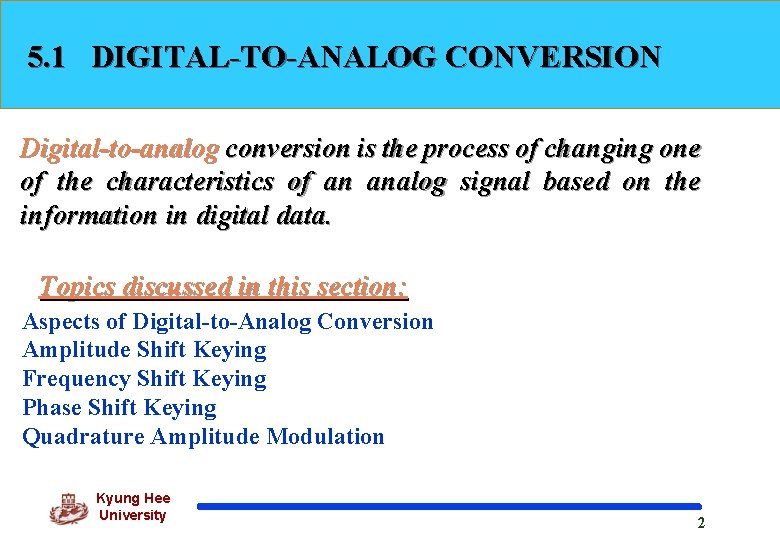5. 1 DIGITAL-TO-ANALOG CONVERSION Digital-to-analog conversion is the process of changing one of the characteristics of an analog signal based on the information in digital data. Topics discussed in this section: Aspects of Digital-to-Analog Conversion Amplitude Shift Keying Frequency Shift Keying Phase Shift Keying Quadrature Amplitude Modulation Kyung Hee University 2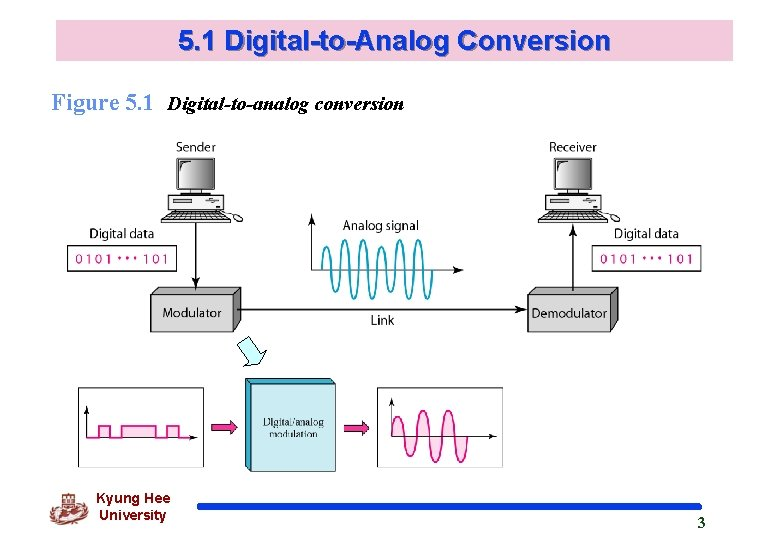5. 1 Digital-to-Analog Conversion Figure 5. 1 Digital-to-analog conversion Kyung Hee University 3Digital-to-Analog Conversion (cont’d) q Type of Digital-to-Analog encoding Kyung Hee University 4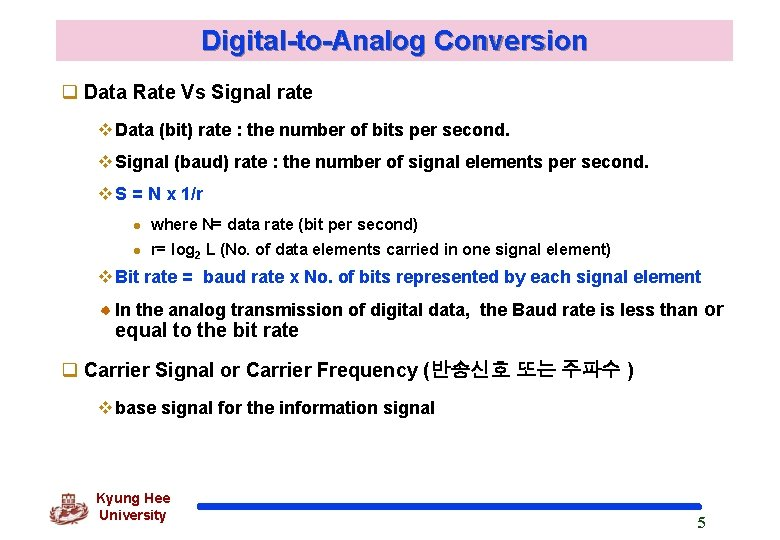Digital-to-Analog Conversion q Data Rate Vs Signal rate v Data (bit) rate : the number of bits per second. v Signal (baud) rate : the number of signal elements per second. v S = N x 1/r l where N= data rate (bit per second) l r= log 2 L (No. of data elements carried in one signal element) v Bit rate = baud rate x No. of bits represented by each signal element In the analog transmission of digital data, the Baud rate is less than or equal to the bit rate q Carrier Signal or Carrier Frequency (반송신호 또는 주파수 ) v base signal for the information signal Kyung Hee University 5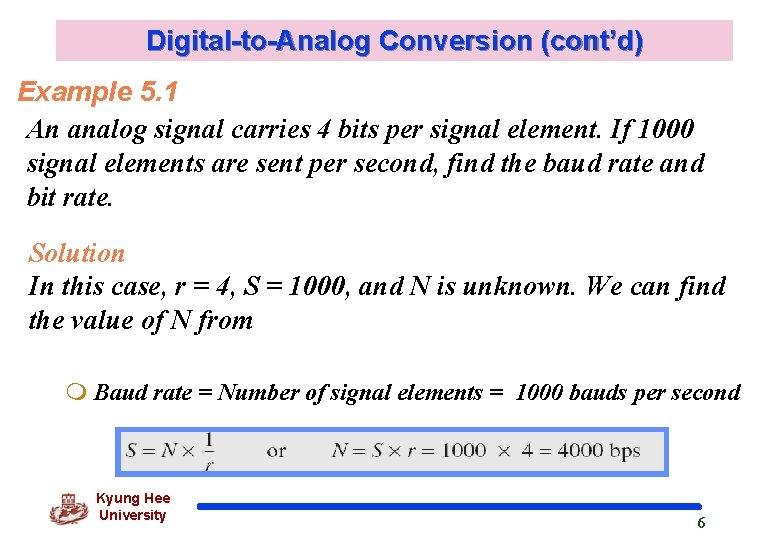Digital-to-Analog Conversion (cont’d) Example 5. 1 An analog signal carries 4 bits per signal element. If 1000 signal elements are sent per second, find the baud rate and bit rate. Solution In this case, r = 4, S = 1000, and N is unknown. We can find the value of N from m Baud rate = Number of signal elements = 1000 bauds per second Kyung Hee University 6Digital-to-Analog Conversion (cont’d) Example 5. 2 An analog signal has a bit rate of 8000 bps and a baud rate of 1000 baud. How many data elements are carried by each signal element? How many signal elements do we need? Solution In this example, S = 1000, N = 8000, and r and L are unknown. We find first the value of r and then the value of L. Kyung Hee University 7Digital-to-Analog Conversion - ASK q. ASK(Amplitude Shift Keying) The amplitude of the carrier signal is varied to create signal element. Both frequency and phase remain constant while the amplitude changes. Highly susceptible to noise interference Figure 5. 3 Binary amplitude shift keying Kyung Hee University 8Digital-to-Analog Conversion - ASK Bandwidth for ASK q Although the carrier signal is only one simple sine wave, the process of modulation produces a nonperiodic composite signal. q Relationship between Signal rate and Bandwidth in ASK B (Bandwidth) = (1 + d) x S l l S : Signal rate (baud) d : factor related to the modulation and filtering process (value of d is between 1 & 0) =S Kyung Hee University Maximum Bandwidth = 2 S 9Digital-to-Analog Conversion - ASK Figure 5. 4 Implementation of binary ASK Kyung Hee University 10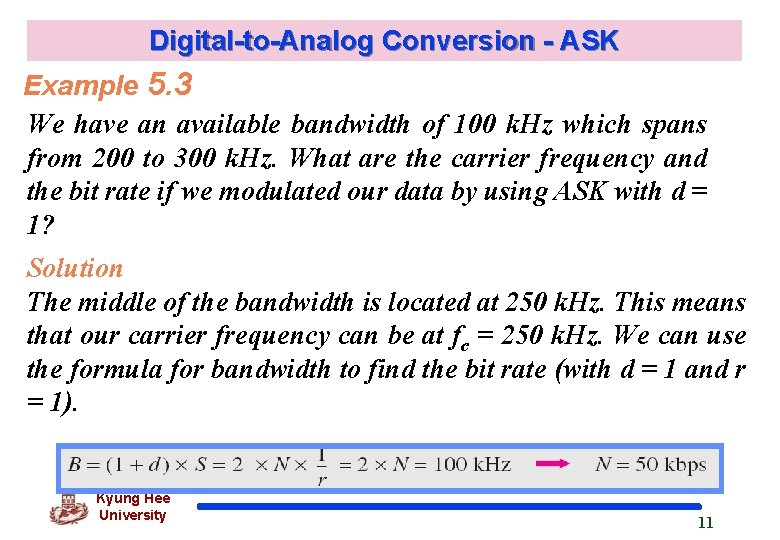Digital-to-Analog Conversion - ASK Example 5. 3 We have an available bandwidth of 100 k. Hz which spans from 200 to 300 k. Hz. What are the carrier frequency and the bit rate if we modulated our data by using ASK with d = 1? Solution The middle of the bandwidth is located at 250 k. Hz. This means that our carrier frequency can be at fc = 250 k. Hz. We can use the formula for bandwidth to find the bit rate (with d = 1 and r = 1). Kyung Hee University 11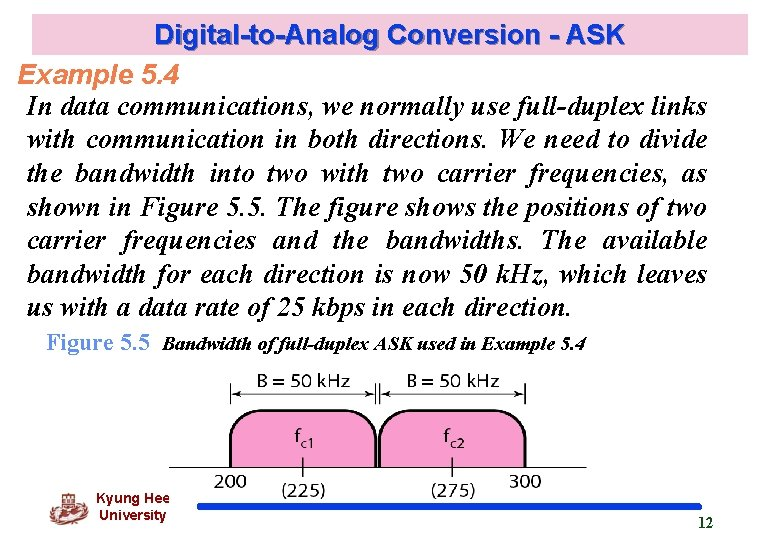Digital-to-Analog Conversion - ASK Example 5. 4 In data communications, we normally use full-duplex links with communication in both directions. We need to divide the bandwidth into two with two carrier frequencies, as shown in Figure 5. 5. The figure shows the positions of two carrier frequencies and the bandwidths. The available bandwidth for each direction is now 50 k. Hz, which leaves us with a data rate of 25 kbps in each direction. Figure 5. 5 Bandwidth of full-duplex ASK used in Example 5. 4 Kyung Hee University 12Digital-to-Analog Conversion - FSK q. FSK(Frequency Shift Keying) the frequency of the carrier signal is varied to represent binary 1 or 0. (Peak amplitude and phase remain constant) Figure 5. 6 Binary frequency shift keying l Both f 1 & f 2 are Δf apart from the midpoint between the two bands. Kyung Hee University l The difference between the two frequency is 2Δf 13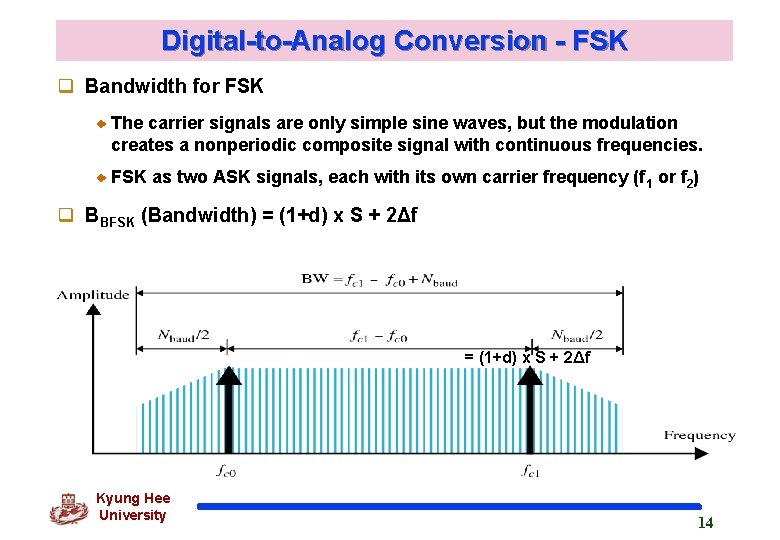Digital-to-Analog Conversion - FSK q Bandwidth for FSK The carrier signals are only simple sine waves, but the modulation creates a nonperiodic composite signal with continuous frequencies. FSK as two ASK signals, each with its own carrier frequency (f 1 or f 2) q BBFSK (Bandwidth) = (1+d) x S + 2Δf Kyung Hee University 14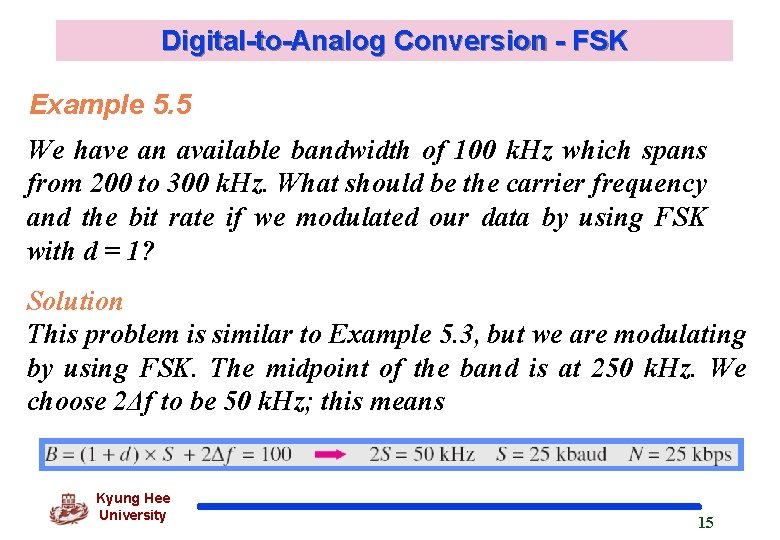Digital-to-Analog Conversion - FSK Example 5. 5 We have an available bandwidth of 100 k. Hz which spans from 200 to 300 k. Hz. What should be the carrier frequency and the bit rate if we modulated our data by using FSK with d = 1? Solution This problem is similar to Example 5. 3, but we are modulating by using FSK. The midpoint of the band is at 250 k. Hz. We choose 2Δf to be 50 k. Hz; this means Kyung Hee University 15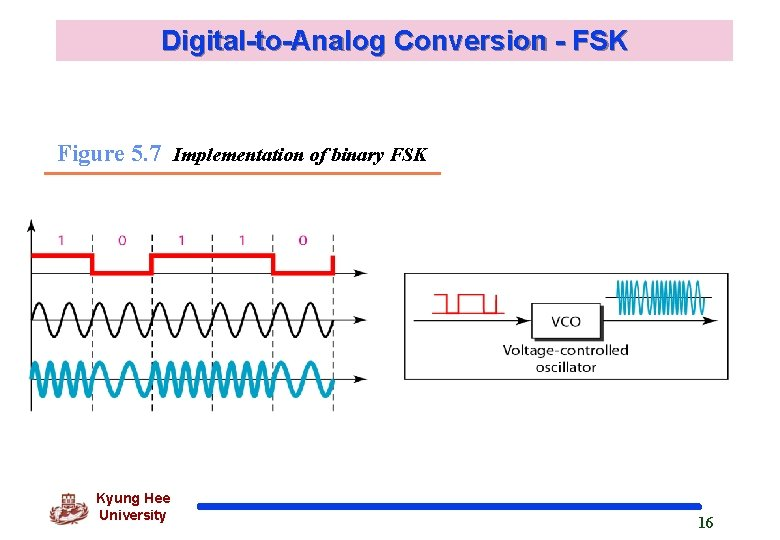Digital-to-Analog Conversion - FSK Figure 5. 7 Implementation of binary FSK Kyung Hee University 16Digital-to-Analog Conversion - FSK • MFSK : Multilevel FSK q We can send data 2 -bits at a time by using 4 frequencies. q BMFSK (Bandwidth) = (1+d) x S + (L-1)2Δf => L x S The minimum value of 2Δf should be at least S for the properation of modulation and demodulation. Kyung Hee University 17Digital-to-Analog Conversion - FSK Example 5. 6 We need to send data 3 bits at a time at a bit rate of 3 Mbps. The carrier frequency is 10 MHz. Calculate the number of levels (different frequencies), the baud rate, and the bandwidth. Solution We can have L = 23 = 8. The baud rate is S = 3 MHz/3 = 1 Mbaud. This means that the carrier frequencies must be 1 MHz apart (2Δf = 1 MHz). The bandwidth is B = 8 × 1000 = 8000 KHz. Figure 5. 8 shows the allocation of frequencies and bandwidth. Kyung Hee University 18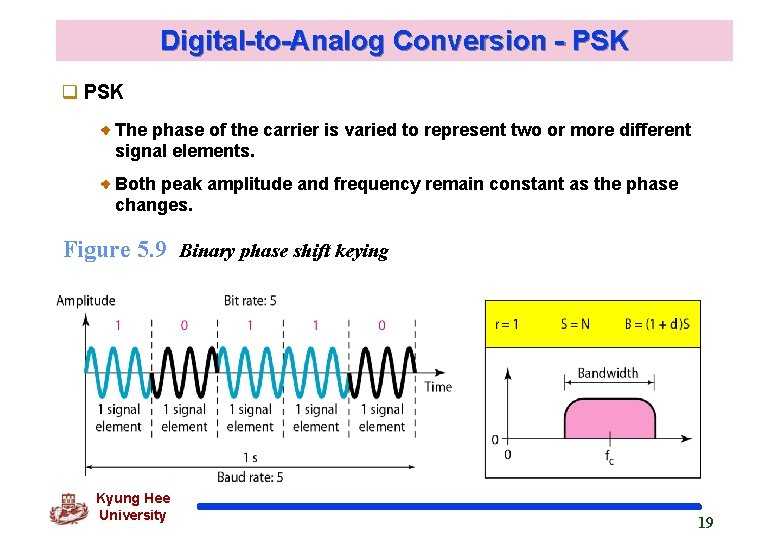Digital-to-Analog Conversion - PSK q PSK The phase of the carrier is varied to represent two or more different signal elements. Both peak amplitude and frequency remain constant as the phase changes. Figure 5. 9 Binary phase shift keying Kyung Hee University 19Relationship between baud rate and bandwidth in PSK q The bandwidth is the same as that for binary ASK, but less than that for BFSK. + Kyung Hee University 20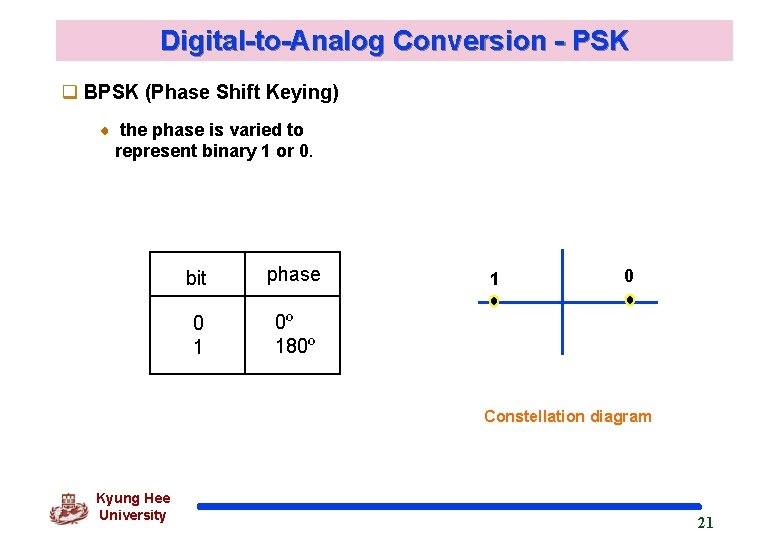Digital-to-Analog Conversion - PSK q BPSK (Phase Shift Keying) the phase is varied to represent binary 1 or 0. bit phase 0 1 0º 180º 1 0 Constellation diagram Kyung Hee University 21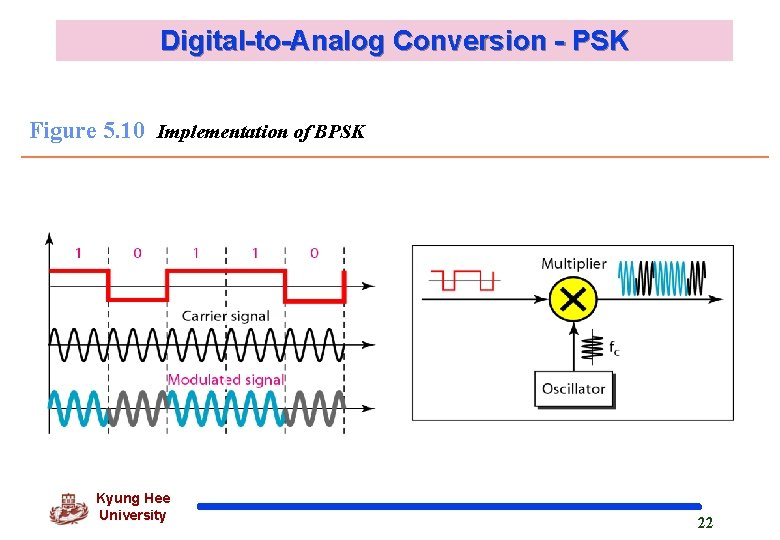Digital-to-Analog Conversion - PSK Figure 5. 10 Implementation of BPSK Kyung Hee University 22QPSK(4 -PSK) method q Instead of utilizing only two variations of a signal, We can use 4 variations and let each phase shift represent 2 bits. q This technique is called 4 -PSK or Q-PSK. Kyung Hee University 23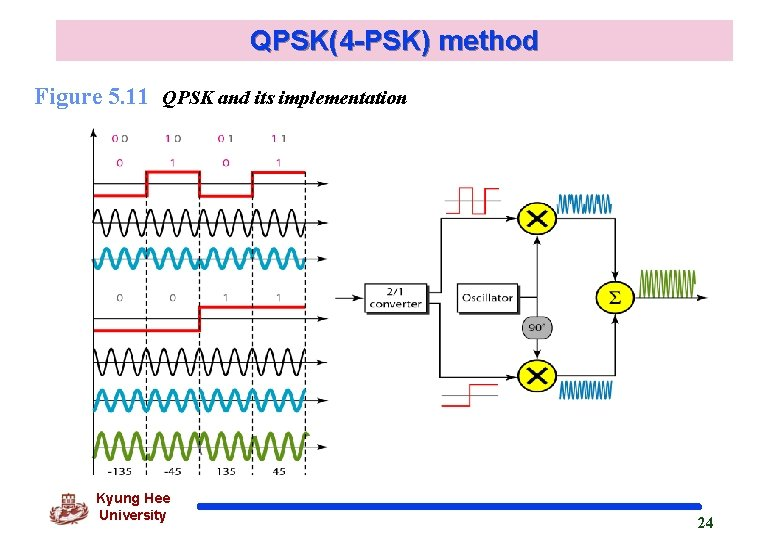QPSK(4 -PSK) method Figure 5. 11 QPSK and its implementation Kyung Hee University 24QPSK(4 -PSK) method Example 5. 7 Find the bandwidth for a signal transmitting at 12 Mbps for QPSK. The value of d = 0. Solution For QPSK, 2 bits is carried by one signal element. This means that r = 2. So the signal rate (baud rate) is S = N × (1/r) = 6 Mbaud. With a value of d = 0, we have B = S = 6 MHz. Kyung Hee University 25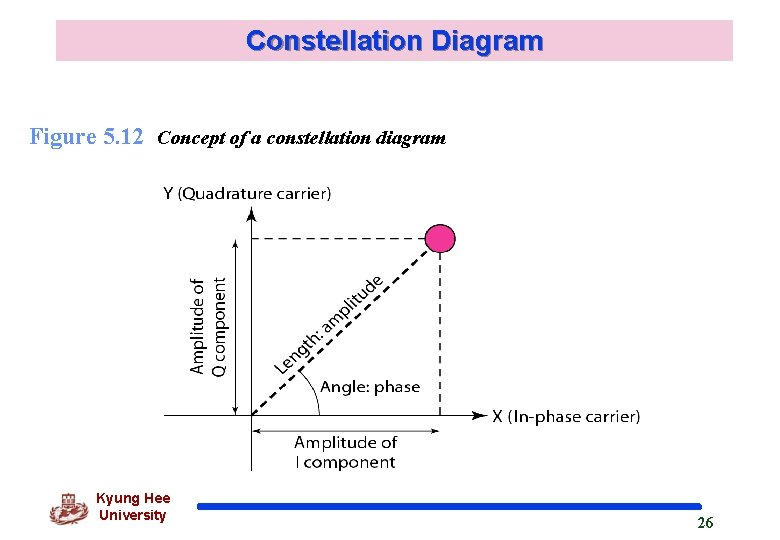Constellation Diagram Figure 5. 12 Concept of a constellation diagram Kyung Hee University 26Constellation Diagram Example 5. 8 Show the constellation diagrams for an ASK (OOK), BPSK, and QPSK signals. Solution Figure 5. 13 shows the three constellation diagrams. Kyung Hee University 27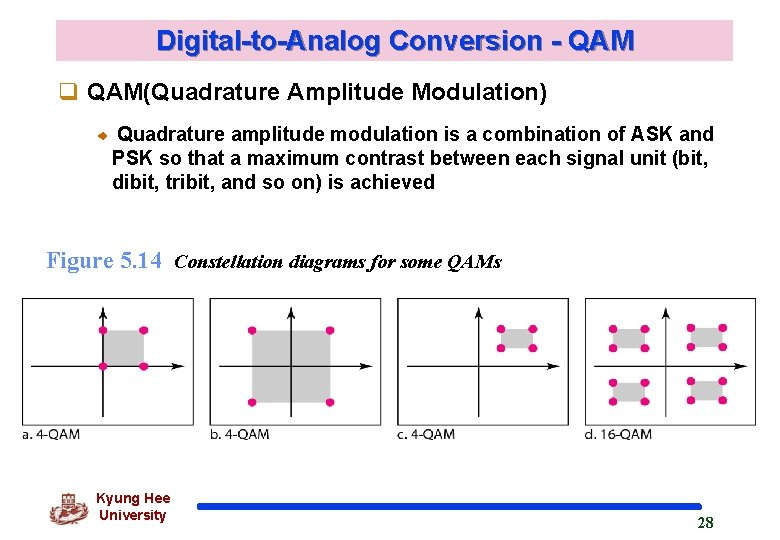Digital-to-Analog Conversion - QAM q QAM(Quadrature Amplitude Modulation) Quadrature amplitude modulation is a combination of ASK and PSK so that a maximum contrast between each signal unit (bit, dibit, tribit, and so on) is achieved Figure 5. 14 Constellation diagrams for some QAMs Kyung Hee University 285. 2 ANALOG-TO-ANALOG CONVERSION Analog-to-analog conversion is the representation of analog information by an analog signal. One may ask why we need to modulate an analog signal; it is already analog. Modulation is needed if the medium is bandpass in nature or if only a bandpass channel is available to us. Topics discussed in this section: Amplitude Modulation Frequency Modulation Phase Modulation Kyung Hee University 29Analog-to-analog Conversion q Analog-to-Analog encoding is the representation of analog information by an analog signal. q Analog-to-Analog encoding Kyung Hee University 30Analog-to-Analog Conversion Figure 5. 15 Types of analog-to-analog modulation Kyung Hee University 31Analog-to-Analog Conversion - AM q. AM(Amplitude Modulation) ~ The frequency and phase of the carrier remain the same; only the amplitude changes to follow variations in the information. Figure 5. 16 Amplitude modulation Kyung Hee University 32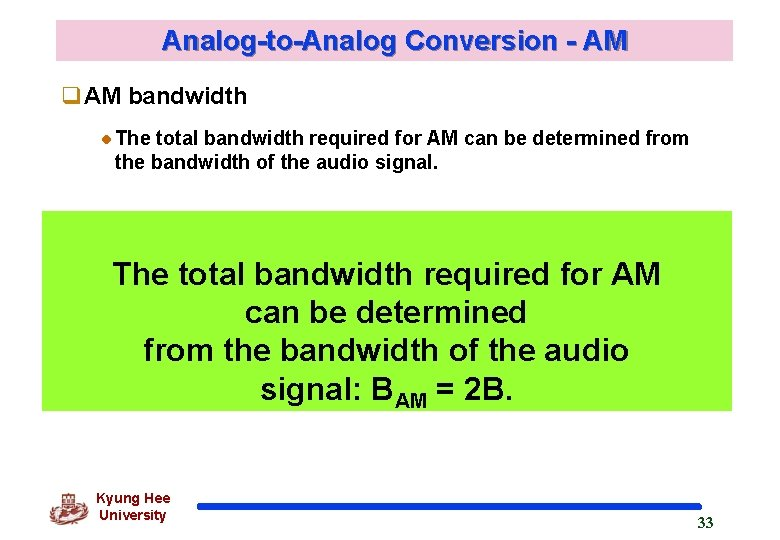Analog-to-Analog Conversion - AM q. AM bandwidth The total bandwidth required for AM can be determined from the bandwidth of the audio signal: BAM = 2 B. Kyung Hee University 33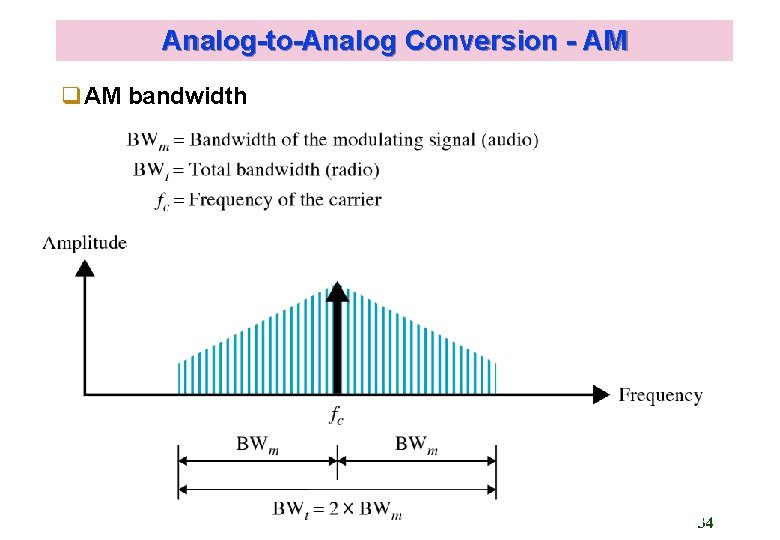Analog-to-Analog Conversion - AM q. AM bandwidth Kyung Hee University 34Analog-to-Analog Conversion - AM q Audio signal(음성과 음악) bandwidth : 5 KHz q Minimum bandwidth : 10 KHz (bandwidth for AM radio station) q AM stations are allowed carrier frequencies anywhere between 530 and 1700 KHz(1. 17 MHz) q each frequency must be separated by 10 KHz Kyung Hee University 35Analog-to-Analog Conversion - AM q AM band allocation Kyung Hee University 36Analog-to-Analog Conversion - FM q. FM(Frequency Modulation) as the amplitude of the information signal changes, the frequency of the carrier changes proportionately. Figure 5. 18 Frequency modulation Kyung Hee University 37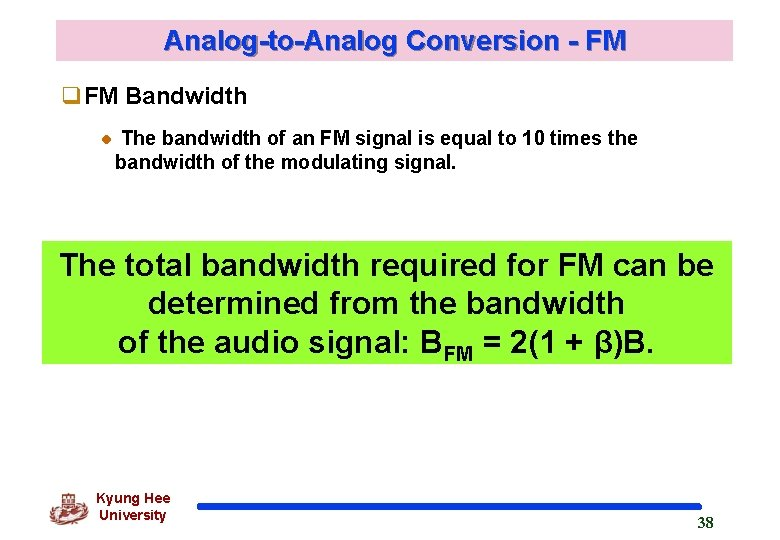Analog-to-Analog Conversion - FM q. FM Bandwidth The bandwidth of an FM signal is equal to 10 times the bandwidth of the modulating signal. The total bandwidth required for FM can be determined from the bandwidth of the audio signal: BFM = 2(1 + β)B. Kyung Hee University 38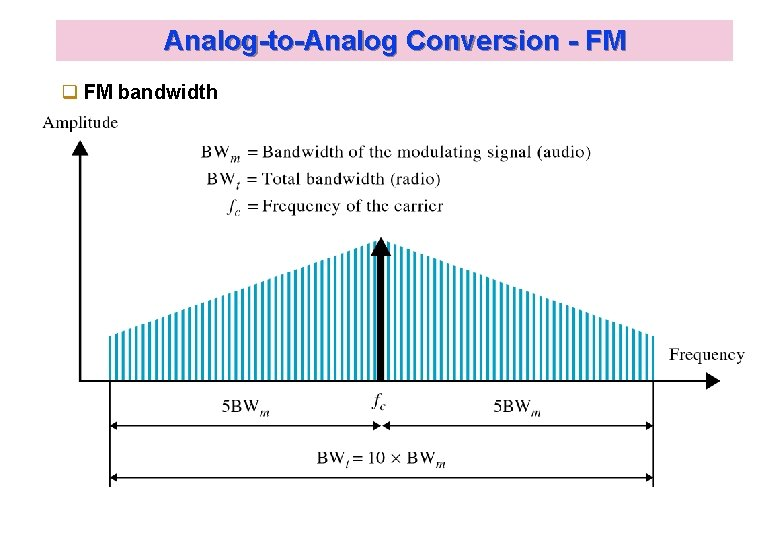Analog-to-Analog Conversion - FM q FM bandwidth Kyung Hee University 39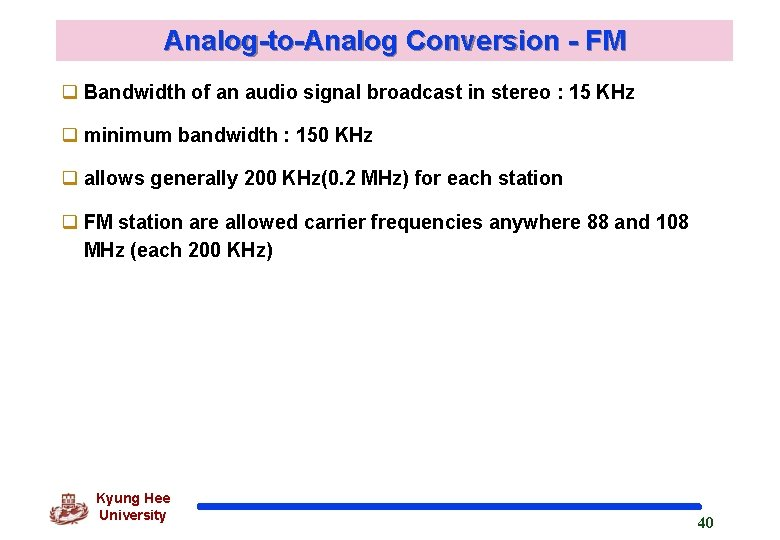Analog-to-Analog Conversion - FM q Bandwidth of an audio signal broadcast in stereo : 15 KHz q minimum bandwidth : 150 KHz q allows generally 200 KHz(0. 2 MHz) for each station q FM station are allowed carrier frequencies anywhere 88 and 108 MHz (each 200 KHz) Kyung Hee University 40Analog-to-Analog Conversion - FM q FM band allocation Alternate bandwidth allocation Kyung Hee University 41Analog-to-Analog Conversion - PM q. PM(Phase Modulation) The phase of the carrier signal is modulated to follow the changing voltage (amplitude) of the modulating signal ~ is used in some systems as an alternative to frequency modulation. Figure 5. 20 Phase modulation Kyung Hee University 42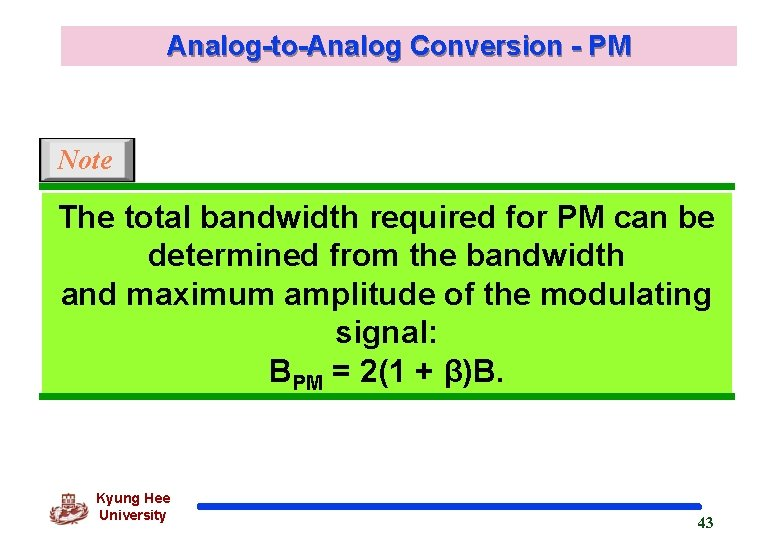Analog-to-Analog Conversion - PM Note The total bandwidth required for PM can be determined from the bandwidth and maximum amplitude of the modulating signal: BPM = 2(1 + β)B. Kyung Hee University 43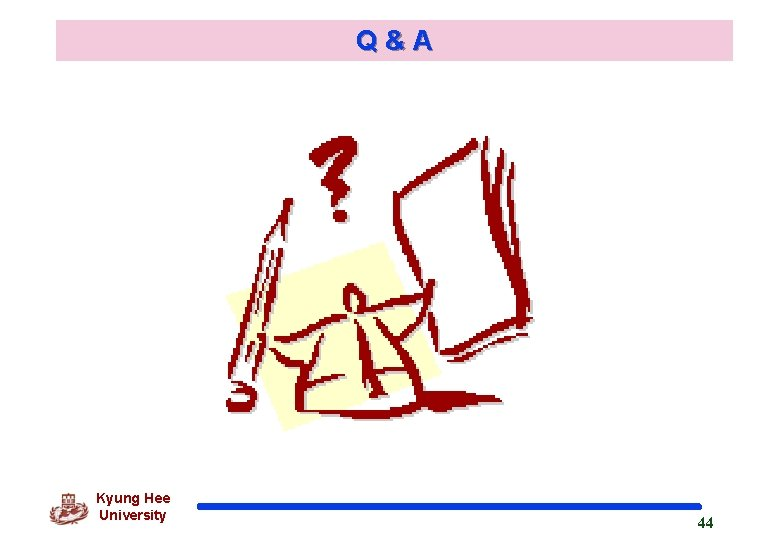Q&A Kyung Hee University 44